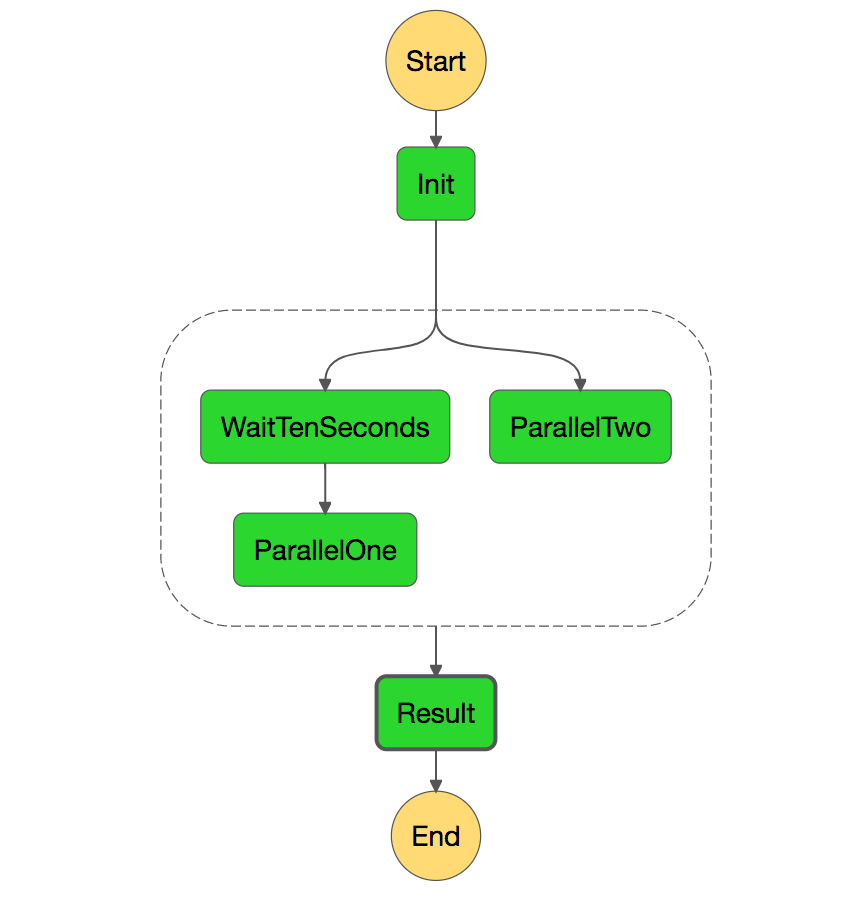In the last article we’ve seen how to create a very basic step function using .Net Core and the serverless framework. Today we’ll see how to create one of the more useful states in a Step Function: the parallel state.

The parallel state allows you to create parallel branches of execution in your state machine. Using it, you’ll be able to run several tasks in parallel and then collect the results in another task, that will be executed only if all the parallel tasks finish correctly.

The task that collects the results of the parallel tasks will receive an array with all the results. The only limitation we have using .Net Core is that the results must be of the same type, so we can have an array of that type. I imagine you can do fancier things using Javascript.

## What are we going to code

We are going to code the following state functionAs you can see we’re going to have an initial function that makes a simple transformation of the input, two parallel functions and a function that collects the results.

## Coding the lambdas

Following the steps of the previous article, create four lambdas called InitLambda, ParallelOneLambda, ParallelTwoLambda and ResultLambda with the following code:

### InitLambda

``````public class InitLambda
{
public string Init(string input){
return \$"Start processing {input}";
}
}
``````

### ParallelOneLambda

``````public class ParallelOneLambda
{
public ParallelResult ParallelOne(string request)
{
return new ParallelResult(DateTime.UtcNow, \$"Parallel one output: {request}");
}
}

public class ParallelResult
{
public ParallelResult(DateTime dateTime, string result)
{
DateTime = dateTime;
Result = result;
}

public DateTime DateTime {get; set;}
public string Result {get;set;}
}
``````

### ParallelTwoLambda

``````public class ParallelTwoLambda
{
public ParallelResult ParallelTwo(string request)
{
return new ParallelResult(DateTime.UtcNow, \$"Parallel two output: {request}");
}
}

public class ParallelResult
{
public ParallelResult(DateTime dateTime, string result)
{
DateTime = dateTime;
Result = result;
}

public DateTime DateTime {get; set;}
public string Result {get;set;}
}

// You can have the result class in a shared library
``````

### ResultLambda

``````public class ResultLambda
{
public string Result(ParallelResult[] input){
return \$"The result of the first task is {input.Result} and the result of the second task is {input.Result}";
}
}

public class ParallelResult
{
public ParallelResult(DateTime dateTime, string result)
{
DateTime = dateTime;
Result = result;
}

public DateTime DateTime {get; set;}
public string Result {get;set;}
}

// You can have the result class in a shared library
``````

As you can see, the result lambda receives an array or ParallelResult.

## Creating the step function

Now it’s time to create the step function. The code is very similar to our original code, but we’re now introducing a new kind of state. Let’s put here the interesting bits:

``````stepFunctions:
stateMachines:
testParallelStepFunction:
definition:
StartAt: Init
States:
Init:
Resource: arn:aws:lambda:\${opt:region}:\${self:custom.accountId}:function:\${self:custom.initService}-\${opt:stage}-Init
Next: ParallelProcessing
ParallelProcessing:
Type: Parallel
Branches:
- StartAt: ParallelOne
States:
ParallelOne:
Resource: arn:aws:lambda:\${opt:region}:\${self:custom.accountId}:function:\${self:custom.parallelOneService}-\${opt:stage}-ParallelOne
End: true
- StartAt: ParallelTwo
States:
ParallelTwo:
Resource: arn:aws:lambda:\${opt:region}:\${self:custom.accountId}:function:\${self:custom.parallelTwoService}-\${opt:stage}-ParallelTwo
End: true
Next: Result
Result:
Resource: arn:aws:lambda:\${opt:region}:\${self:custom.accountId}:function:\${self:custom.resultService}-\${opt:stage}-Result
End: true
``````

As you can see we have a new task state called ParallelProcessing which is of type Parallel and has two branches: ParallelOne and ParallelTwo. The branches follow the state machine definition and, in this case, have only a task inside them.

We can now deploy the lambdas and the step function and invoke it:

``````sls invoke stepf --name testParallelStepFunction --data '"asdf"'
``````

And see that we have the expected result:## A couple of remarks

### A branch can be a state machine by itself

A branch is not limited to have just one task inside it. It can have several tasks. The only limitation is that the last task must have a final one (have `End:true`)### The results always come in order

The order of the results in the array on the step after a parallel step always come in the same order, and that order is the order that the branches are defined. So, although ParallelOne ends later than ParallelTwo, the result of ParallelOne will come in the first position of the array.

## Summary

We’ve seen one of the more powerful states of a State Function, the parallel step. We’ve seen how we can add more than one task inside each of its branches and how to collect the results.